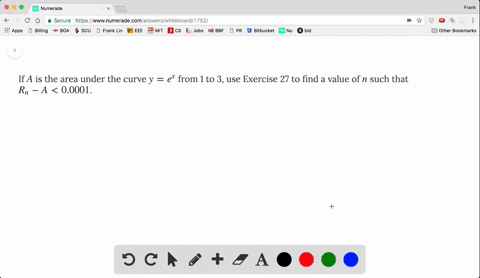### If $A$ is the area under the curve $y = e^x$ …

01:04
FL
Problem 27

Let $A$ be the area under the graph of an increasing continuous function $f$ from $a$ to $b$, and let $L_n$ and $R_n$ be the approximations to $A$ with $n$ subintervals using left and right endpoints, respectively.

(a) How are $A$, $L_n$, and $R_n$ related?

(b) Show that $$R_n - L_n = \frac{b - a}{n} [f(b) - f(a)]$$
Then draw a diagram to illustrate this equation by showing that the $n$ rectangles representing $R_n - L_n$ can be reassembled to form a single rectangle whose area is the right side of the equation.

(c) Deduce that $$R_n - A < \frac{b - a}{n} [f(b) - f(a)]$$

(A). $L_{n} \leq A \leq R_{n}$
(B). See explanation for result.
(C). $R_{n}-A<\frac{b-a}{n}[f(b)-f(a)]$

## Discussion

You must be signed in to discuss.

## Video Transcript

So here we have increasing function and we try to on a say exact area under the curve and his left and point rectangles. Are these Orion Point on Iraq tangos Because it's increasing function. Ryan Point always overestimate, and the left end point always underestimate us. Eleven point the smallest one and Ryan Point is there biggest one on the interval. Improved thiss. So the idea is this. We write on the formula for using left and pointed right and point to estimate as we've seen earlier problems and former look site. The attacks Time Some I from verses hero to a minus one F off a plus i b minus a over. You know, I think off this B minus a over and rectangle. Basically, we takes Assemble point. The first one's a second one's a prostate. I extend my access. This quality that's their wines f off a press to their attacks and for right on point is this. From one to again, we take the symbol point. The first one's a plus Delta X seven wise a plus two kayaks, except the last one is a process and Delta X or a Z or F off beat. So what is difference between these two? We have fact around this B minus a over on which keeps us this part Good. And how about the difference between the some mention? Well, with some of this, this one, if you think of it, is f off a plus Delta X up to f off a plus. Andale taiex a press and the attacks happened to be. And how about this one? This one We start with F or a bills. The first one we use the left and point which is says the left end of the interval, which is a and the last one's eff off a plus a minus one day with my ex. So what happened is the difference between these two. We cancel the terms in the middle if we do the subtraction, because all sorry. Oh, if we do this one minus this one, we'LL cancel the term in the middle. Except the term that's not repeats on FM point. We happen to use this term we don't use in right employee and Ryan Point. We used this term which we don't use in the left hand points. So we are left with half off this minor set off this and this haven't we be so if you laugh with f off, be myself off a here now leave you to trawl the diagram and deduced this. And this is kind of obvious because Bill's days between this and if the difference of these two it's given by this quantity, then the wine the middle should be closer to to the r and compared to the other one. So this difference will be smaller than I remind you that pride and size are minus era and this is kind of trivial from the relation giving the party.